# Python program to find greatest integer using floor() method

An example of floor() method in Python: Here, we are going to learn how to find the greatest integer using floor() method in Python?
Submitted by Anuj Singh, on August 06, 2019

The greatest integer function is a function (real numbers function) to itself that is defined as follows: it sends any real number to the largest integer that is less than or equal to it.

The greatest integer function of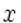is denoted by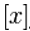.

The greatest integer function is related to the fractional part (sometimes denoted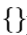) of the number as follows: for any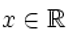, we have: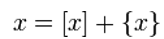More about greatest integer function: floor() and ceiling functions | wikipedia

## Python code to find greatest integer (Use of floor() method)

```# Python code to find greatest integer
# (Use of floor() method)

import math #importing class

num = float(input("Enter any float number: "))
print("math.floor(num): ", math.floor(num))

num = float(input("Enter another float number: "))
print("math.floor(num): ", math.floor(num))
```

Output

```Enter any float number: 56.892
math.floor(num):  56
Enter another float number: -34.567
math.floor(num):  -35
```

Preparation